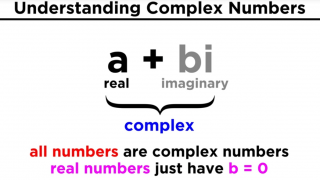trustle > EngliSea > Corona Safe Schooling > mathematics > Quick Math Index > 30 Complex Number

The set C of complex numbers is the set R2 of ordered pairs of real numbers. a+bi = [a,b]

For 2 dimensional numbers,
x = [p∠φ] = [p·→φ,p·↑φ] = [a,b]
y = [q∠ψ] = [q·→ψ,q·↑ψ] = [c,d]

Multiplication x·y = [(p·q)∠(φ+ψ)]
= [(p·q)·→(φ+ψ),(p·q)·↑(φ+ψ)]
= [p·q·(→φ·→ψ−↑φ·↑ψ), p·q·(↑φ·→ψ+→φ·↑ψ)]
= [p·→φ·q·→ψ−p·↑φ·q·↑ψ, p·↑φ·q·→ψ+p·→φ·q·↑ψ]
= [a·c−b·d,  ...Complex Number
Hamilton introduced the approach to define the set C of complex numbers as the set R2 of ordered pairs [a,b] of real numbers and [a,b] + [c,d] = [a+c,b+d] [a,b] · [c,d] = [ac-bd,bc+ad] It is then just a matter of notation to express [a,b] as a+bi.
9609Dave
9608e, mathematical constant
e = (1+1/n)⩘n「n⪫∞」= Σ(1/n!)「n⪫∞」
9668-